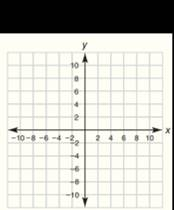Chapter 10.CT, Problem 5CTElementary Geometry For College St...

7th Edition
Alexander + 2 others
ISBN: 9781337614085

Solutions

Chapter
SectionElementary Geometry For College St...

7th Edition
Alexander + 2 others
ISBN: 9781337614085
Textbook Problem

Complete the following table of x and y-coordinates of points on the graph of the equation 2 x + 3 y = 12 . x 0 3 9 y 4To determine

Complete the following table by using the equation 2x+3y=12.

Explanation

Substitute the given values in the equation thus find the unknown values.

Substitute x=0 in the given equation 2x+3y=12,

20+3y=12

On simplifying,

3y=12

The coefficient of y is 3, thus divide both sides by 3.

3y3=123

y=4

Thus the first point is 0, 4.

Substitute x=3 in the given equation 2x+3y=12,

23+3y=12

On simplifying,

6+3y=12

3y=12-6

3y=6

The coefficient of y is 3, thus divide both sides by 3.

3y3=63

y=2

Thus the second point is 3, 2

Still sussing out bartleby?

Check out a sample textbook solution.

See a sample solution

The Solution to Your Study Problems

Bartleby provides explanations to thousands of textbook problems written by our experts, many with advanced degrees!

Get Started

In Exercises 2340, find the indicated limit. 33. limx22x+1x+2

Applied Calculus for the Managerial, Life, and Social Sciences: A Brief Approach

Logarithmic Form Write the equation in logarithmic form. 25. 26 = 64

Precalculus: Mathematics for Calculus (Standalone Book)

Subtract 51229.

Contemporary Mathematics for Business & Consumers

In Exercise 69 and 70, find curl (FxG)=x(FxG) F(x,y,z)=i+3xj+2yk G(x,y,z)=xiyj+zk

Calculus: Early Transcendental Functions (MindTap Course List)

True or False: If converges and converges, then converges.

Study Guide for Stewart's Multivariable Calculus, 8th